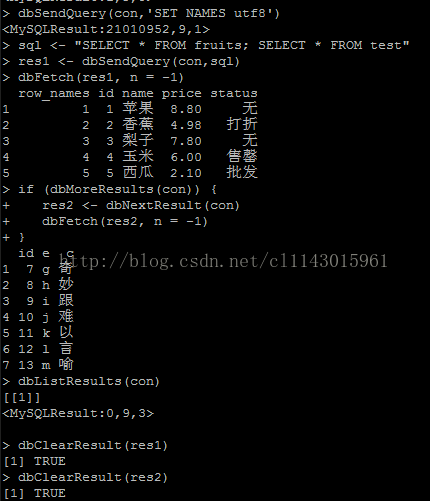## R语言使用RMySQL连接及读写Mysql数据库_r语言 dbname-程序员宅基地

``````install.packages("RMySQL")
library(RMySQL)
help(package="RMySQL") #查看说明文档
#创建数据库连接
con <- dbConnect(MySQL(), host="", dbname="", user="", password="")``````
``````summary(con) #获取连接信息
dbGetInfo(con) #获取连接信息
dbListTables(con) #查看database下所有表格
dbRemoveTable(con, "test") #删除名为test的表``````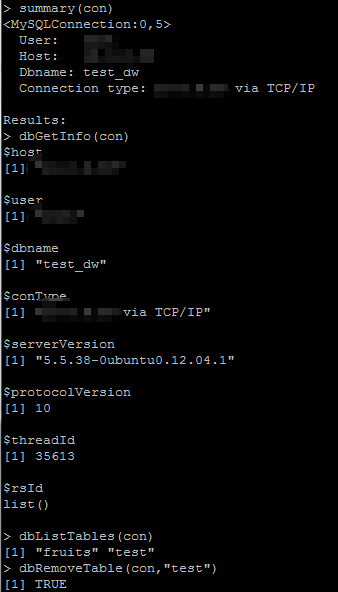#写数据库表

``````#写数据库表
fruits <-data.frame(id=1:5,name=c("苹果","香蕉","梨子","玉米","西瓜"),price=c(8.8,4.98,7.8,6,2.1),status=c("无","打折","无","售罄","批发"))
dbListTables(con)
dbWriteTable(con,"fruits",fruits)
dbListTables(con)
``````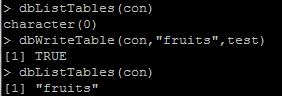#读数据库

``````#读数据库
dbSendQuery(con,'SET NAMES utf8')``````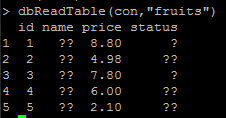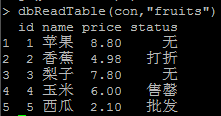#写数据表：直接/追加/覆盖

``````#写数据表，覆盖追加
testA <-data.frame(id=1:6,e=c("a","b","c","d","e","f"),c=c("我","的","世","界","变","得"))
testB <-data.frame(id=7:13,e=c("g","h","i","j","k","l","m"),c=c("奇","妙","跟","难","以","言","喻"))
#直接写testA写入test表中
dbWriteTable(con,"test",testA,row.names=F)
#追加写testB追加在test表后
dbWriteTable(con,"test",testB,append=T,row.names=F)
#覆盖写testB覆盖test表
dbWriteTable(con,"test",testB,overwrite=T,row.names=F)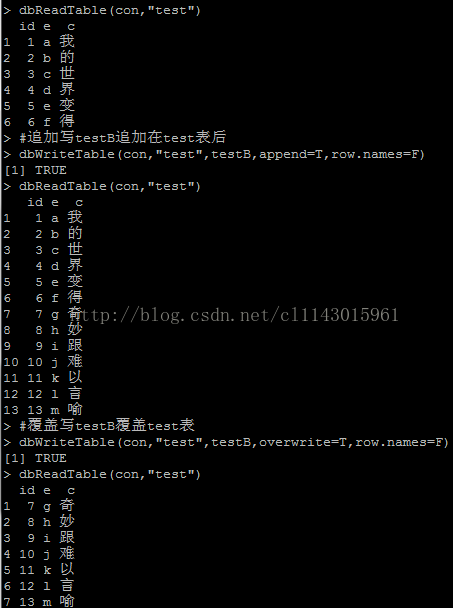#用SQL语句查询结果

``````#用SQL语句查询dbGetQuery()和dbSendQuery()两种方法

#直接获得查询结果
dbGetQuery(con, "SELECT * FROM fruits limit 3")

#给查询结果赋值
res <- dbSendQuery(con, "SELECT *FROM fruits")
#获取前2条数据，n=-1时是获取所有数据
data <- dbFetch(res, n=2)
#再获取余下几条数据
data <- dbFetch(res, n=-1) #取余下所有数据
#清楚查询结果
dbClearResult(res)
#断开连接
dbDisconnect(con)``````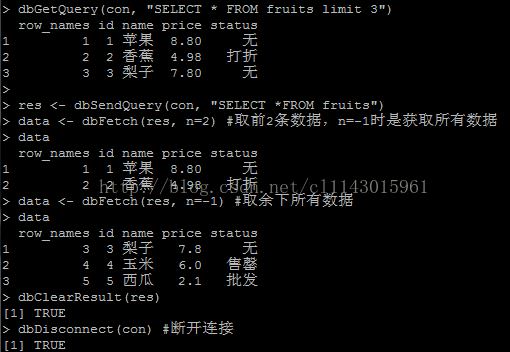#用SQL语句批量查询

``````#用SQL语句批量查询
dbSendQuery(con,'SET NAMES uft-8')
sql <- "SELECT * FROM fruits;SELECT * FROM test"
res1 <- dbSendQuery(con,sql)
dbFetch(res1, n = -1)
if (dbMoreResults(con)) {
res2 <- dbNextResult(con)
dbFetch(res2, n = -1)
}
dbListResults(con)
dbClearResult(res1)
dbClearResult(res2)

dbDisconnect(con)
``````